# Multi Objective Optimization of a Power Distribution System Based on Mixed Integer Programming

Multi Objective Optimization of a Power Distribution System Based on Mixed Integer Programming

Ndjependa Patrik Roger Boum Alexandre TeplairaNdjakomo Essiane Salomé

University of Douala, Douala, P.O. Box 1872, Cameroon

Corresponding Author Email:
teplaira.boum@ubuea.cm
Page:
39-46
|
DOI:
https://doi.org/10.18280/jesa.530105
21 September 2019
|
Accepted:
6 December 2019
|
Published:
29 February 2020
| Citation

OPEN ACCESS

Abstract:

This paper proposes a multi objective optimization of a power distribution system. The technique used here is network reconfiguration. The goal is to find an optimal configuration of the network which minimizes power losses while avoiding voltage drops and overloads online. To attain that goal, we minimize the active power losses and the voltage deviation at each node of the network. The minimization of the voltage deviation here consists in minimizing the reactive power losses online. The tool used is the GAMS software. The problem is solved by Mixed Integer Programming (MIP) which is a deterministic method. This method is implemented on two standard IEEE test power distribution networks 33-node and 69-node. The results obtained are satisfactory compared to other techniques in the literature.

Keywords:

multi objective optimization, power distribution system, reconfiguration, mixed integer programming

1. Introduction

Electric energy is a product of everyday consumption which is characterized by a certain number of parameters such as: current, voltage, power, energy, consumption, etc. The role of power transmission and distribution lines is to transport energy from production sites to consumption sites, with steps to lower the voltage level in transformer stations.

In the literature, several studies have been carried out on minimizing online power losses in a power distribution system. In the field of optimization, there are metaheuristic or approximate methods and exact or deterministic methods.

Boum et al.  proposed a reconfiguration of the 33-node IEEE test network using the SOS algorithm. It turns out that the execution time is low and active power losses are minimized compared to other algorithms such as the genetic algorithm. They propose a study which is limited to the standard network IEEE 33-node and consequently the results are not reliable to conclude from the efficiency of the algorithm.

Wang and Cheng  proposed an optimization of a 33-node IEEE power distribution network by reconfiguration by applying the Plant Growth Simulation Algorithm (PGSA). They do not take into account the improvement in the voltage profile at the network nodes.

Nguyen and Thruong  proposed a CSA algorithm (Cukoo Search algorithm). The authors presented a reconfiguration of the 33-node and 69-node IEEE networks minimizing active power losses online and improving the voltage profile. They propose a method which does not clearly explain the technique used to minimize the voltage deviation at the level of the nodes.

Rayapudi et al.  proposed an HSA (Harmony Search Algorithm) method and applied it on a 33-node network. The results show an optimal optimal reconfiguration.

Muttaqi et al.  proposes an algorithm which determines the power losses for the different combinations of possible switches and selects the one which has the least power losses. He compares these results with those of other methods. They have only applied their algorithm on an IEEE 33- node network which is insufficient to conclude from the effectiveness of a method.

Niknam  proposed a reconfiguration based on an evolutionary algorithm which is the combination of Honey Bee Mating Optimization and the Discrete Particle Swarm Optimization (DPSO) called DPSO-MBMO. This algorithm is implemented and compared to other methods.

Zhen-kun et al.  proposed a Hybrid Particle Swarm Optimization which combines binary PSO and Discrete PSO. This technique is used for the problem of optimal reconfiguration. The authors first apply the binary PSO which selects a group of branches which should be opened. Second, they implement the second DPSO algorithm which selects the branch that should be opened in the branch group. This method converges quickly and has good stability.

Li et al.  proposed a PSO hybrid combining the binary PSO and the Discrete PSO. The results obtained show that this algorithm is robust and converges quickly.

Khodr et al.  propose a reconfiguration of the power distribution network formulated as a Mixed Integer Nonlinear Programming (MINLP) problem with a nonlinear objective function with binary decision variables. Difficulties encountered in solving nonlinear optimization problems with binary decision variables force the authors to use partition techniques such as Benders decomposition. Benders' partition algorithm is a two-level decomposition technique, master and slave, which defines an iterative procedure between two ordered levels for the search for the optimal solution. The master level in their work, represents the decision problem which is defined as a MINLP problem while the slave level deals with the operations problem, being a nonlinear OPF problem. This method allows us an appropriate treatment of the non-convexity associated with the binary variables and divides the total problem in two under problems easy to solve. The master problem determines the new network configuration and the open switches. This problem is solved by the branch and bound method using the CPLEX solver. This solution obtained by CPLEX is transferred to the slave sub-problem which checks the technical feasibility of the solution of the master problem. The problem is solved using the CONOPT solver. They do not study the improvement of the tension profile.

The reconfiguration of the power distribution network aims to choose a switching combination of branches of the network which optimizes certain performance criteria of the system while maintaining certain constraints. The ability to automatically reconfigure the network quickly and reliably is a key to the requirement for Smart Grid .

Hyder and Mahata  presents a reconfiguration of the distribution network using the binary programming method. The mathematical formulation of the problem is a quadratic form and solved using the QMIP (Quadratic Mixed Integer Programming) algorithm. This technique applies to IEEE 32 bus, 70 bus, 135 bus standard distribution networks. The solver used for solving the mathematical problem is GUROBI.

Abdulaziz et al.  formulate a model for planning the distribution of power from an electrical network to solve the sizing, timing and location of the distribution station and the problems of expansion of the supply lines simultaneously. The objective function of the model represents the present value of the investment costs and energy losses of the system which occur throughout the planning period. The objective function (cost value) is minimized with the following constraints: power limits, avoiding voltage drops. The resolution algorithm used is MILP (Mixed Integer Linear Programming). They must propose results which must first be validated on a standard IEEE network.

Recent developments in optimal power flow (OPF) for radial networks open the promise of greater sophisticated management of power distribution networks. Such sophistication is necessary to effectively exploit the new dynamic energy resources. However, to be useful, optimization tools must also take into account the management of preexisting technologies which involve discrete decision variables .

Briglia et al.  propose methods to include discrete decision variables on transformer tracks and capacitor banks as well as ON / OFF loads. The algorithms used are MILP (Mixed Integer Linear Programming) with CIR (Convex Integer Relaxations). The simulation results obtained are applied to an electrical energy distribution network in Uruguay.

Krengel et al.  present an approach of examining the city's districts as a whole in order to create a basis for investment decisions relating to the supply of energy to residential housing on the basis of an optimized conversion of distributed energy. Using MILP (Mixed Integer Linear Programming), an optimal configuration of energy conversion units such as heat pumps, photovoltaic systems or conventional heating systems is determined taking into account the investment as well as their costs. Operation over a period of 20 years. In addition, it is possible to design a district heating network using graph theory to take advantage of load balancing effects and economies of scale. The functionality of the model is illustrated by the analysis of a small-town district in Germany.

Koutsoukis et al.  use a hybrid non-linear method. They propose a planning method for distribution networks which determines the optimal network reinforcement and expansion plan taking into account the service factors provided by decentralized production units. The proposed method calculates network investments and operational costs in order to meet future demand load as well as the connection of new loads and decentralized production units. Results on a 21-bus power distribution system validates the performance of the method.

Borghetti  discusses minimum power losses, the problem of configuration of power distribution networks, including generation integrated by the MILP method. A model is proposed which takes into account the operating constraints typical of distribution networks, connection intensity limits and bus voltage requirements as well as the presence of integrated generation. The precision of the results and the calculation performance of the project are proposed.

In this paper, Mixed Integer Programming is proposed to find an optimal configuration of a power distribution system that minimizes the active and reactive power losses and therefore the voltage deviation at the nodes of the system.

The proposed method is very effective. It is a deterministic method which seeks the exact solution. This algorithm is implemented on two standard IEEE networks (33-node and 69-node), which justifies the reliability of the method. The results obtained are better compared to other algorithms in the literature.

2. Problem Formulation

Consider the single-line diagram of a line shown in Figure 1.

## 1.jpg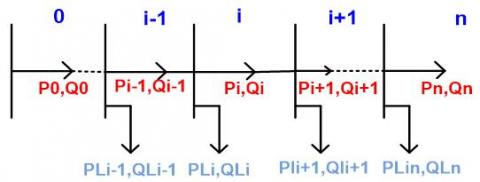Figure 1. Single-line diagram of the line

Figure 1 shows a simplified single-line diagram of a part of an electrical power distribution network comprising branches and nodes. The customers are connected at the node level and are represented by their active and reactive powers.

The objective is to minimize line power losses by reconfiguring the distribution network. The minimization of the reactive power losses leads to the minimization of the voltage deviation at each node of the network.

The objective function of the problem is formulated as follows:

$\min F=\min \left(f_{1}, f_{2}\right)$    (1)

f1: Total active losses power (Ploss).

f2: Total reactive losses power (Qloss).

$f_{1}=P_{l o s s}$

$f_{2}=Q_{\text {loss}}$

We then obtain the following function F:

$\min F=\alpha f_{1}+\beta f_{2}$    (2)

$\alpha$ and $\beta$ are the weighting coefficients.

Contraints

During reconfiguration, it is necessary to avoid voltage drops and overloads at each node. So, you need the constraints for the objective function. They are:

$V_{i \min } \leq V_{i} \leq V_{i \max } ; i=0,1,2, \ldots, N_{b u s}-1$    (3)

$0 \leq I_{i} \leq I_{\text {imax}} ; i=0,1,2, \ldots, N_{b r}-1$    (4)

With:

Vi, min: Minimum value of the acceptable voltage at a node

Vi, max: Maximum value of the acceptable voltage at a node

Ii: Intensity of line current flowing through a branch

Ii, max: Intensity of the maximum line current defined by the manufacturer

Nbr: Number of  branch

Nbus: Number of node

For the calculation of the power flow we use the following relationships:

$P_{i+1}=P_{i}-r_{i} \frac{P_{i}^{2}+Q_{i}^{2}}{V_{i}^{2}}-P_{L i+1}$    (5)

$Q_{i+1}=Q_{i}-x_{i} \frac{P_{i}^{2}+Q_{i}^{2}}{V_{i}^{2}}-Q_{L i+1}$    (6)

$V_{i+1}^{2}=V_{i}^{2}-2\left(r_{i} P_{i}+x_{i} Q_{i}\right)+\left(r_{i}^{2}+x_{i}^{2}\right) \frac{P_{i}^{2}+Q_{i}^{2}}{V_{i}^{2}}$    (7)

With:

Pi: Active power at node i

Qi: réactive power at node i

Pi+1: Active power at node i+1

Qi+1: Réactive power at node i+1

ri: Resistance of the branch i

xi: Reactance of the branch i

Vi: RMS value of the voltage at node i

Vi+1: RMS value of the voltage at node i i +1

PLi+1: Active power of the load connected at node i +1

QLi+1: Reactive power of the load connected at node i +1

The total active power losses are expressed by the Eq. (8).

$P_{\text {loss}}=\sum_{i=0}^{n-1} \frac{P_{i}^{2}+Q_{i}^{2}}{V_{i}^{2}} r_{i}$    (8)

n=Nbr

The total reactive power losses are expressed by the Eq. (9).

$Q_{\text {loss}}=\sum_{i=0}^{n-1} \frac{P_{i}^{2}+Q_{i}^{2}}{V_{i}^{2}} x_{i}$    (9)

The objective of the reconfiguration is to minimize the active and reactive power losses of during the power transit, the problem is formulated as follows:

$\min F=\alpha \sum_{i=0}^{n-1} \frac{P_{i}^{2}+Q_{i}^{2}}{V_{i}^{2}} r_{i}+\beta \sum_{i=0}^{n-1} \frac{P_{i}^{2}+Q_{i}^{2}}{V_{i}^{2}} x_{i}$    (10)

The reconfiguration must obey the following rules:

·         All loads must be supplied with electrical energy (if not the majority)

·         The network configuration (power transit) must be radial.

·         The network is linear.

In this study, the vector of decision variables giving the state of the switches is:

$Y=\left[y_{1} y_{2} y_{3} y_{4} y_{5} \dots y_{N b r}\right]$

After binary coding, the following state of the switches is obtained by branch:

$S=\left[S_{1} S_{2} S_{3} S_{4} S_{5} \ldots S_{N b r}\right]$

3. Problem Solving Algorithm

In this paper, the methodology for solving the problem involves calculating the power flow of the network.

Description of the algorithm

Step 1: Initialization

This step defines the number of switches (branches) of the network and the objective functions.

Step 2: Declaration of parameters and decision variables

This step is used to declare the optimization parameters, variables and the binary branch connection decision variables.

Step 3: Network configuration

This step is used to configure the network and the characteristics of the lines and loads connected at the nodes are specified.

Step 4: Calculation of the voltages at the nodes of the initial configuration

After configuration, the voltages of each node are determined (initial configuration).

Step 5: Calculation of the active and reactive power losses of the initial configuration

Power losses are calculated using the recurrence equations for calculating power flow.

Step 6: Calculation of the voltage deviation at the nodes of the initial configuration

The highest voltage deviation of the network is determined.

Step 7: Minimize active power losses

This phase makes it possible to find the optimum of the objective function using Mixed Integer Programming (MIP).

Step 8: Minimize the losses of reactive power

This step allows you to find the optimum of the objective function using Mixed Integer Programming (MIP).

Step 9: Calculation of the voltage deviation of the new network configuration

The voltages are determined at the nodes of the configuration which minimizes the power active and reactive losses as well as the voltage deviation.

Step 10: Verification of constraints

Step 11: Show the result

4. Results and Discussion

The program is written in GAMS 23.5. The computer characteristics are: Processor: 1.70 GHz; RAM: 4.00 GB; OS: 64-bit WINDOWS 10.

In this paper, we implement our program on two standard IEEE test networks 33-node and 69-node.

4.1 IEEE test network 33-node

Presentation of the structure:

The problem resolution flowchart is given in Figure 2.

## 2.jpg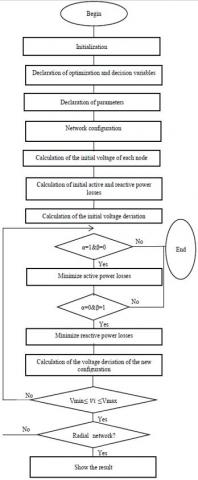Figure 2. Problem resolution flowchart

The structure of the IEEE 33 node network used is given in Figure 3.

## 3.jpgFigure 3. Structure of the IEEE 33 bus

Results

The RMS value of the voltage at node 0 of the network is 12.66 kV. The total active and reactive powers are 3715 kW and 2300 kVAr respectively. These data and the characteristics of this network as well as the powers of the charges are taken in work .

In this study, binary words are read from S1 to S37 and read from left to right.

The following binary code representing the state of the switches before reconfiguration is given in Figure 4.

In implementing the optimization program, the optimal binary reconfiguration code is given in Figure 5.

Table 1 presents the results obtained before and after reconfiguration.

Figure 6 presents the voltage profile before and after reconfiguration by applying the proposed algorithm for IEEE 33-node system.

Table 2 presents a comparative study between the results of the proposed algorithm and those found in the literature for an IEEE 33-node network.

Discussion

Table 1 presents the results before and after reconfiguration of the IEEE 33 bus network. Before the reconfiguration, the open switches are: S33, S34, S35, S36, S37. The active power losses are equal to 211.581 kW, the reactive power losses to 144.548 kVAr and the minimum node voltage is 0.9130 p.u which implies a network voltage deviation of 0.0870 p.u. After reconfiguration by the proposed algorithm, the open switches are S7, S9, S14, S32, S37 and the active power losses online are 136.765 kW, a reduction of 35.36%. The minimum voltage at the node of the network is 0.9348 p.u which implies a voltage deviation is 0.0652 p.u, a reduction of 25.05%.

Figure 6 shows an improvement of the tension profile of the nodes after reconfiguration using the proposed method. Before the reconfiguration, the minimum voltage was equal to 0.9130 p.u and after reconfiguration 0.9348 p.u. which implies a voltage deviation is 0.0652 p.u, a reduction of 25.05%.

These results are compared to those of the literature in Table 2. This comparative study shows that for the proposed algorithm, the minimum active power losses after reconfiguration are equal to 136.765 kW and the minimum voltage is 0.9348 p.u. These results are compared with those of the papers [3, 4] and are better. The results obtained by the HSA show that the minimum active power losses after reconfiguration are equal to 146.39 kW. The difference of power losses with the proposed algorithm is 9.625 kW, a reduction of 6.57%. In addition the minimum voltage obtained is 0.9336 p.u which implies a difference of 0.0012 p.u with the proposed algorithm. The minimum active power losses obtained by the CSA method are equal to 138.87 kW. The difference of power losses with the proposed technique is 2.105 kW, a reduction of 1.51%. The proposed method reduces the minimum losses by 6.57% compared to those of HSA and by 1.51% compared to those of CSA obtained.

## 4.jpgFigure 4. State of the switches for the 33-node system before reconfiguration

## 5.jpg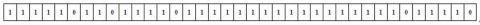Figure 5. State of the switches for the 33-node system after reconfiguration

## 6.jpgFigure 6. Voltage profile for the 33-node system before and after reconfiguration

Table 1. Reconfiguration of the IEEE 33 Bus network

 Before reconfiguration After reconfiguration Reduction(%) Open switches S33, S34, S35, S36, S37 S7, S9, S14, S32, S37 / Real losses power (kW) 211.581 136.765 35.36 Reactive losses power (kVAr) 144.548 35.229 75.62 Vmin (p.u) 0.9130 0.9348 / Vde(p.u) 0.0870 0.0652 25.05

Vmin: Minimal voltage at node; Vde: Voltage deviation

Table 2. Comparative study of the results of other algorithms for a network of 33 bus

 Methods Open switches Power loss Vde (p.u)(kW) Vmin (p.u) Initial S33, S34, S35, S36, S37 211.581 0.0870 0.9130 Proposed method S7, S9, S14, S32, S37 136.765 0.0652 0.9348 CSA  S7, S9, S14, S32, S37 138.87 0.0576 0.94235 PSO  S7, S9, S14, S32, S37 138.87 0.0576 0.94235 HSA  S7, S10, S14, S28, S36 146.39 0.0664 0.9336

4.2 IEEE 69 bus test network

Presentation of the structure

The RMS value of the voltage at node 0 of the network is 12.66 kV. The total active and reactive powers are respectively 3802 kW and 3696 kVAr. The characteristics of this network are the same as those used in work .

Figure 7 shows the structure of the network. While table 3 shows the different switches associated with each branch.

Results

In this study, the binary words are from S1 to S73 and read from left to right. The following binary code representing the state of the switches before reconfiguration is given in Figure 8. In implementing the optimization program, the optimal binary reconfiguration code is given in Figure 9. Table 4 presents the results obtained before and after reconfiguration. Figure 10 presents the voltage profile before and after reconfiguration by applying the proposed algorithm for IEEE 33-node system. Table 5 presents a comparative study between the results of the proposed algorithm and those found in the literature for an IEEE 69-node network.

Table 3. Switches associated with each branch

 Bus to bus Switches Bus to bus Switches Bus to bus Switches 1-2 S1 26-27 S26 51-52 S51 2-3 S2 3-28 S27 52-53 S52 3-4 S3 28-29 S28 53-54 S53 4-5 S4 29-30 S29 54-55 S54 5-6 S5 30-31 S30 55-56 S55 6-7 S6 31-32 S31 56-57 S56 7-8 S7 32-33 S32 57-58 S57 8-9 S8 33-34 S33 58-59 S58 9-10 S9 34-35 S34 59-60 S59 10-11 S10 3-36 S35 60-61 S60 11-12 S11 36-37 S36 61-62 S61 12-13 S12 37-38 S37 62-63 S62 13-14 S13 38-39 S38 63-64 S63 14-15 S14 39-40 S39 64-65 S64 15-16 S15 40-41 S40 11-66 S65 16-17 S16 41-42 S41 66-67 S66 17-18 S17 42-43 S42 12-68 S67 18-19 S18 43-44 S43 68-69 S68 19-20 S19 44-45 S44 11-43 S69 20-21 S20 45-46 S45 13-21 S70 21-22 S21 4-47 S46 15-46 S71 22-23 S22 47-48 S47 50-59 S72 23-24 S23 48-49 S48 27-65 S73 24-25 S24 49-50 S49 25-26 S25 8-51 S50

Table 4. Reconfiguration of the IEEE 69 Bus network

 Before reconfiguration After reconfiguration Reduction(%) Open switches S69, S70, S71, S72, S73 S14, S57, S61, S69, S70 / Real losses power (kW) 224.95 97.917 56.47 Reactive losses power (kVAr) 37.538 23.256 38.04 Vmin (p.u) 0.9100 0.9500 / Vde(p.u) 0.0900 0.0500 44.44

Table 5. Comparative study of the results of other algorithms for a network of 69 bus

 Methods Open switches Power loss Vde (p.u)(kW) Vmin (p.u) Initial S69, S70, S71, S72, S73 224.95 0.0900 0.9100 Proposed method S14, S57, S61, S69, S70 97.917 0.0500 0.9500 CSA  S14, S57, S61, S69, S70 98.5680 0.0505 0.9495 PSO  S14, S57, S61, S69, S70 98.5680 0.0505 0.9495 HSA  S13, S18, S56, S61, S69 99.35 0.0572 0.9428

## 7.jpg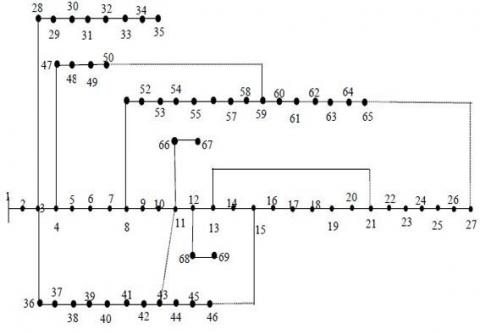Figure 7. Structure of IEEE 69 bus network

## 8.jpg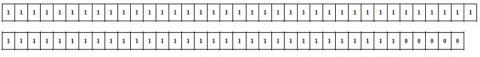Figure 8. State of the switches for the 69-node system before reconfiguration

## 9.jpg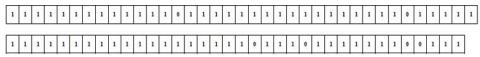Figure 9. State of the switches for the 69-node system after reconfiguration

Discussion

Table 4 presents the results before and after reconfiguration of the IEEE 69 bus network. Before the reconfiguration, the open switches are: S69, S70, S71, S72, S73. The active power losses are equal to 224.95 kW, the reactive power losses to 37.538 kVAr and the minimum node voltage is 0.9100 p.u which implies a network voltage deviation of 0.0900 p.u. After reconfiguration by the proposed algorithm, the open switches are S14, S57, S61, S69, S70 and the active power losses online are 97.917 kW, a reduction of 56.47%. The minimum voltage at the node of the network is 0.9500 p.u which implies a voltage deviation is 0.0500 p.u, a reduction of 44.44%.

Figure 10 shows an improvement in the voltage profile of the nodes after reconfiguration using the proposed method. Before the reconfiguration, the minimum voltage was equal to 0.9100 p.u and after reconfiguration 0.9500 p.u. which implies a voltage deviation is 0.0500 p.u, a reduction of 44.44%.

These results are compared to those of the literature in Table 5. This comparative study shows that for the proposed algorithm, the minimum active power losses after reconfiguration are equal to 97.917 kW and the minimum voltage is 0.9100 p.u. These results are compared with those of the papers [3, 16] and are better. The results obtained by the HSA show that the minimum active power losses after reconfiguration are equal to 99.35 kW. The difference of power losses with the proposed algorithm is 1.433 kW, a reduction of 1.44%. In addition the minimum voltage obtained is 0.9428 p.u which implies a difference of 0.0072 p.u with the proposed algorithm. The minimum active power losses obtained by the CSA method are equal to 98.5680 kW. The difference of power losses with the proposed technique is 0.651 kW, a reduction of 0.66%. The minimum voltage obtained is 0.9495 p.u which implies a difference of 0.0005 p.u with the proposed algorithm. The proposed method reduces the minimum power losses by 1.44% compared to those of the HSA obtained and by 0.66% compared to those of the CSA obtained.

## 10.jpgFigure 10. Profile voltage for the 69-node system before and after reconfiguration

5. Conclusions

A multi objective optimization of an electrical energy distribution network has been studied in this paper. An optimal configuration which minimizes power losses and voltage deviation at network nodes is proposed. The optimization technique used is deterministic and based on Mixed Integer Programming (MIP). This technique is implemented on the IEEE 33 bus and IEEE 69 bus standard networks. The results obtained show that this method is very efficient. A comparative study with other algorithms in the literature confirms the effectiveness of this approach.

References

 Boum, A.T., Ndjependa, P.R., Ngo Bisse, J. (2017). Optimal reconfiguration of power systems based on symbiotic organism search. Journal of Power and Energy Engineering, 5(11): 1-9. https://doi.org/10.4236/jpee.2017.511001

 Wang, C., Cheng, H.Z. (2008). Optimization of network configuration in large distribution systems using plant growth simulation algorithm. IEEE Transactions on Power Systems, 23(1): 119-126. https://doi.org/10.1109/TPWRS.2007.913293

 Nguyen, T.T., Truong, A.V. (2015). Distribution network reconfiguration for power loss minimization and voltage profile improvement using cuckoo search algorithm. Electrical Power and Energy Systems, 68: 233–242. https://doi.org/10.1016/j.ijepes.2014.12.075

 Rayapudi, S.R., Lakshmi, V., Sadhu, N., Manyala, R.R., Rao, A.S. (2011). Optimal network reconfiguration of large-scale distribution system using harmony search algorithm. IEEE Trans Power Syst., 26(3):1080–8. https://doi.org/10.1109/TPWRS.2010.2076839

 Muttaqi, K.M., Ganapathy, V., Jasmon, G.B., Buhari, M. (2000). A novel method for loss minimization in distribution networks. University of Wollongong, Faculty of Engineering and Information Sciences, IEEE, London, UK. https://doi.org/10.1109/DRPT.2000.855672

 Niknam, T. (2000). An efficient hybrid evolutionary algorithm based on PSO and HBMO algorithms for multi-objective distribution feeder reconfiguration. Energy Conversion and Management, 50(8): 2074-2082. https://doi.org/10.1016/j.enconman.2009.03.029

 Li, Z.K., Chen, X.Y., Yu, K., Liu, H.M., Zhao, B. (2008). Hybrid particle swarm optimization for distribution network reconfiguration. Proceedings of the CSEE.

 Li, Z., Chen, X., Yu, K., Sun, Y., Liu, H.M. (2008). A hybrid Particle Swarm Optimization approach for distribution network reconfiguration problem. IEEE Power and Energy Society General Meeting in the 21st Century. https://doi.org/10.1109/PES.2008.4596635

 Khodr, H., Martinez-Crespo, J., Matos, M., Pereira, J. (2009). Distribution systems reconfiguration based on opf using benders decomposition. IEEE Trans Power Deliver, 24(4): 2166-2176. https://doi.org/10.1109/TPWRD.2009.2027510

 Hyder, M.M., Mahata, K. (2016). Reconfiguration of distribution system using a binary programming model. AIMS Energy, 4(3): 461–480. https://doi.org/10.3934/energy.2016.3.461

 Abdulaziz, A.U.M., Alhabib, H.I. (2010). Power networks planning using mixed-integer programming. JKAU: Eng. Sci., 21(2): 15-34.

 Briglia, E., Alaggia, S., Paganini, F. (2017). Distribution network management based on optimal power flow: Integration of discrete decision variables. 51st Annual Conference on Information Sciences and Systems (CISS).

 Krengel, S., Falke, T., Schnettler, A. (2013). Optimization model for the energy supply in city quarters. 22nd International Conference on Electricity Distribution, Stockholm.

 Koutsoukis, N., Georgilakis, P., Hatziargyrioy, N. (2017). Active distribution network planning based on hybrid genetic algorithm-nonlinear programming method. 24th International Conference on Electricity Distribution Glasgow.

 Borghetti, A. (2012). A mixed-integer linear programming approach for the computation of the minimum-losses radial configuration of electrical distribution networks. IEEE Transactions on Power Systems, 27(3): 1264-1273. https://doi.org/10.1109/TPWRS.2012.2184306

 Rao, S.R., Ravindra, K., Satisk K., Narasimham, S.V.L. (2013). Power loss minimization in distribution system using network reconfiguration in the presence of distributed generation. IEEE Trans Power Syst., 28(1): 317-325. https://doi.org/10.1109/TPWRS.2012.2197227